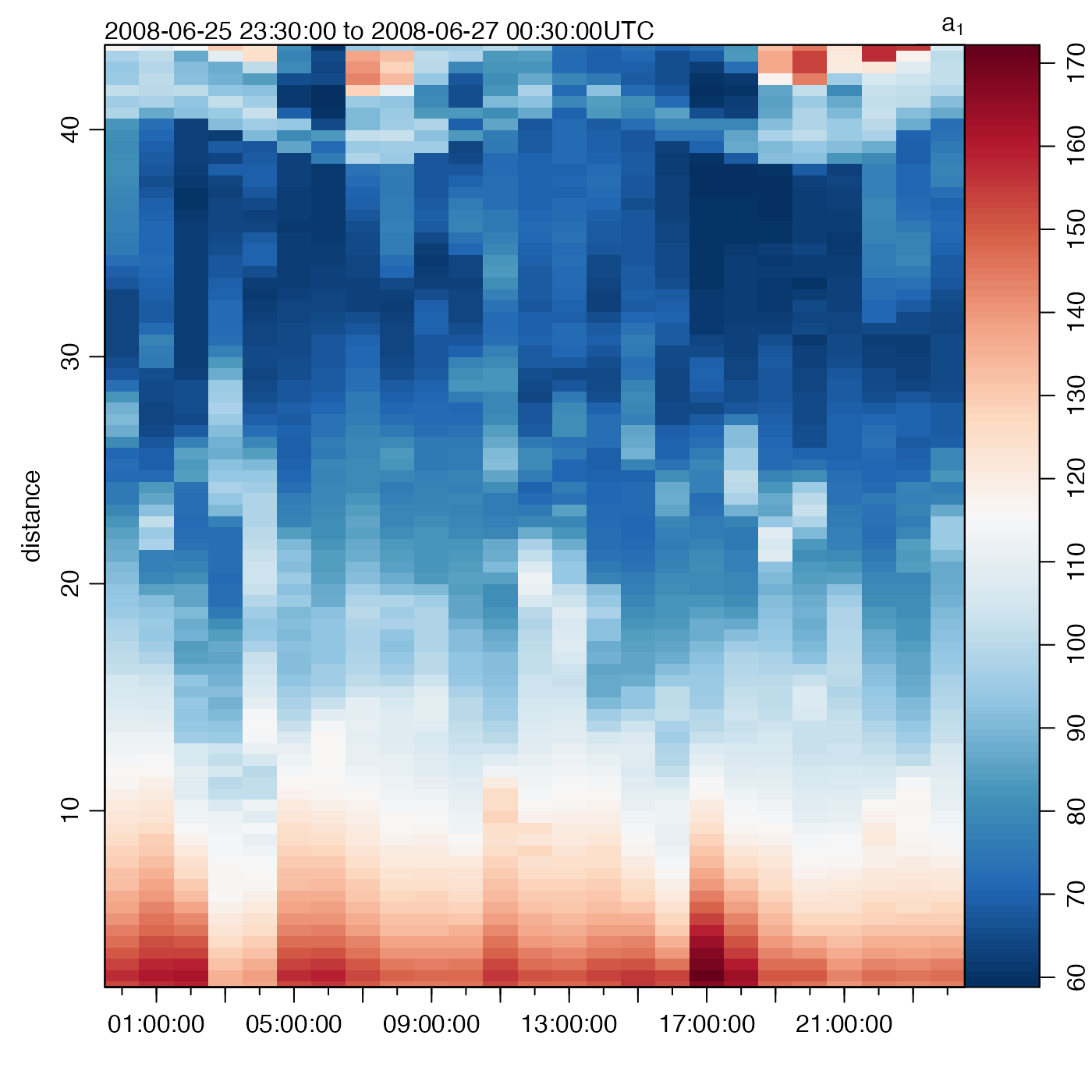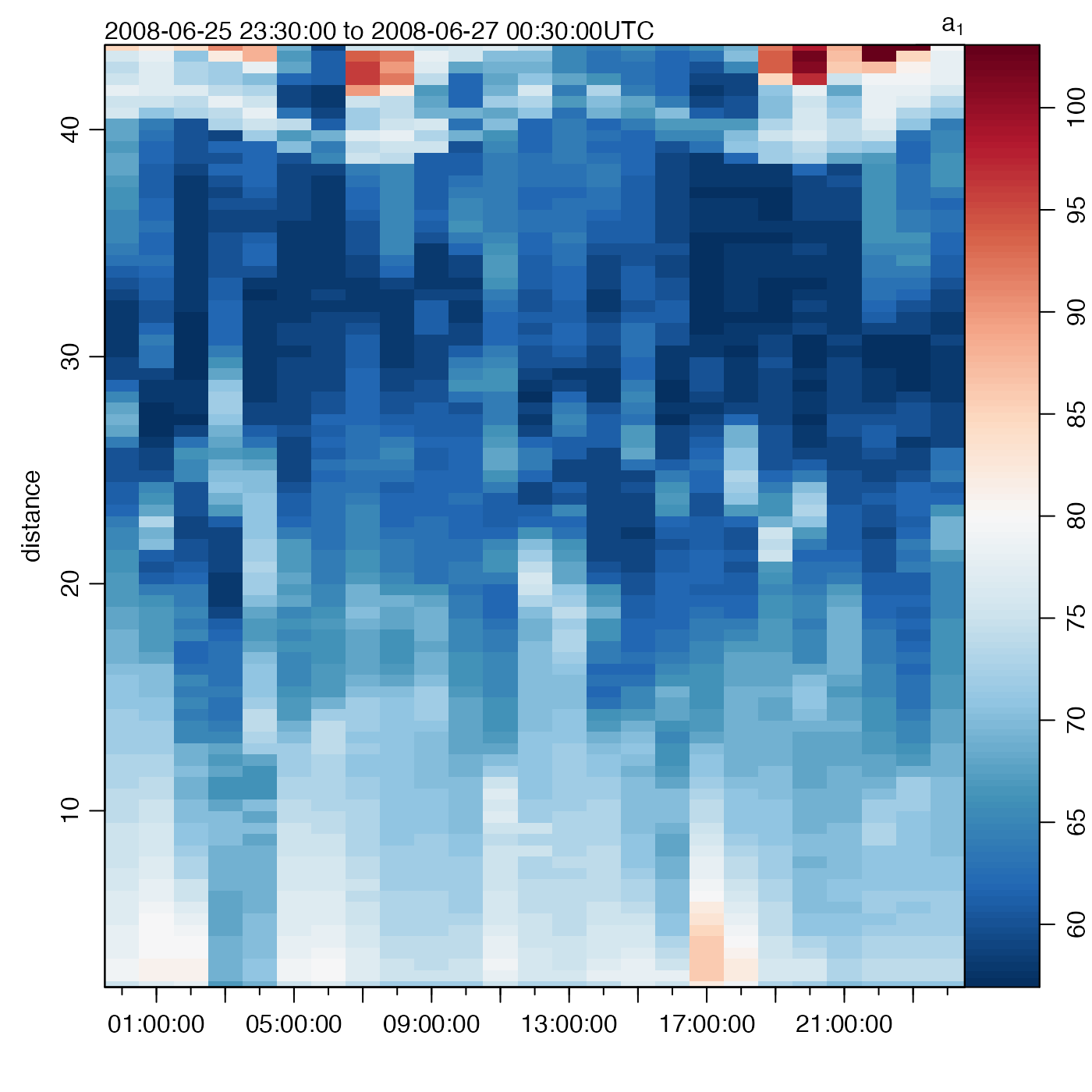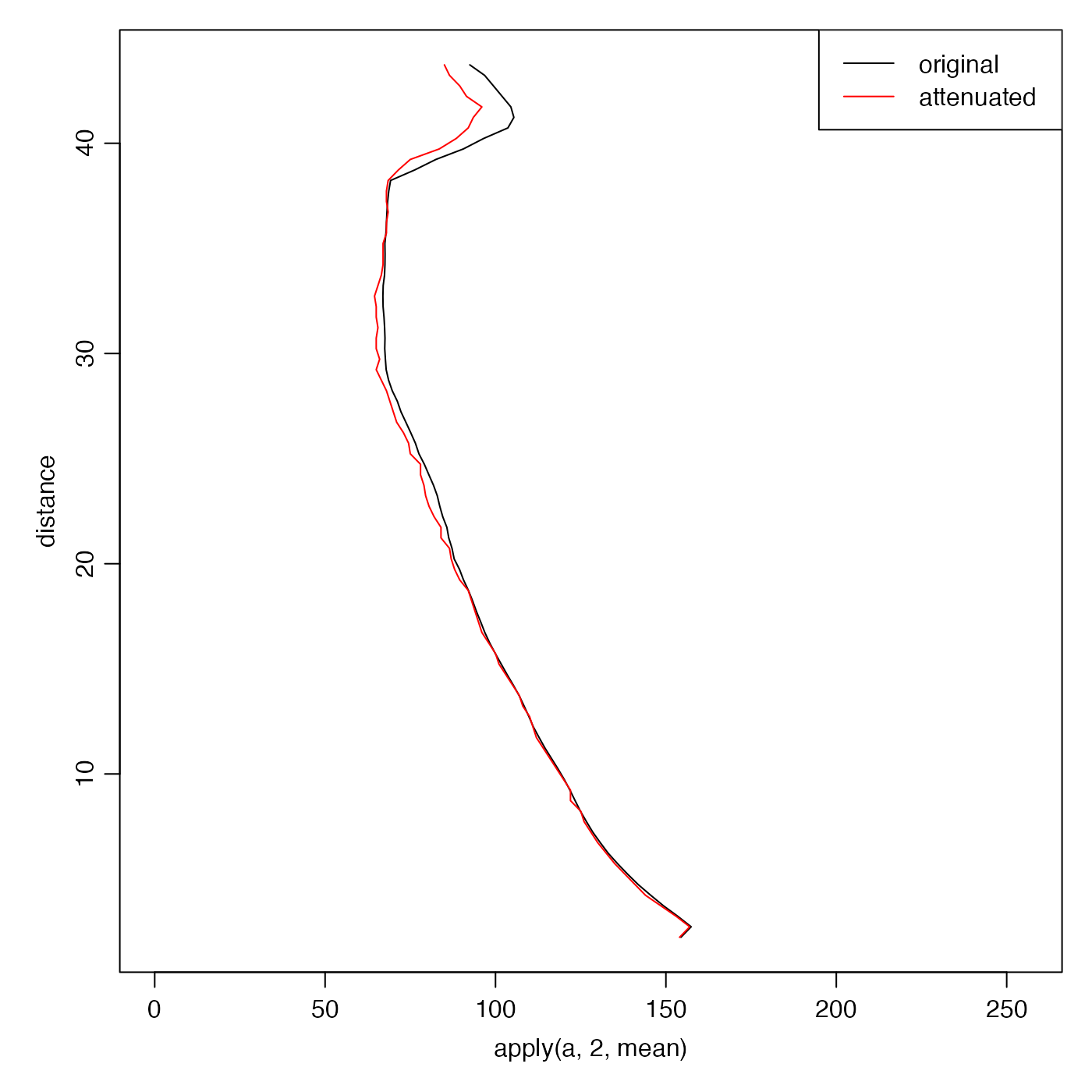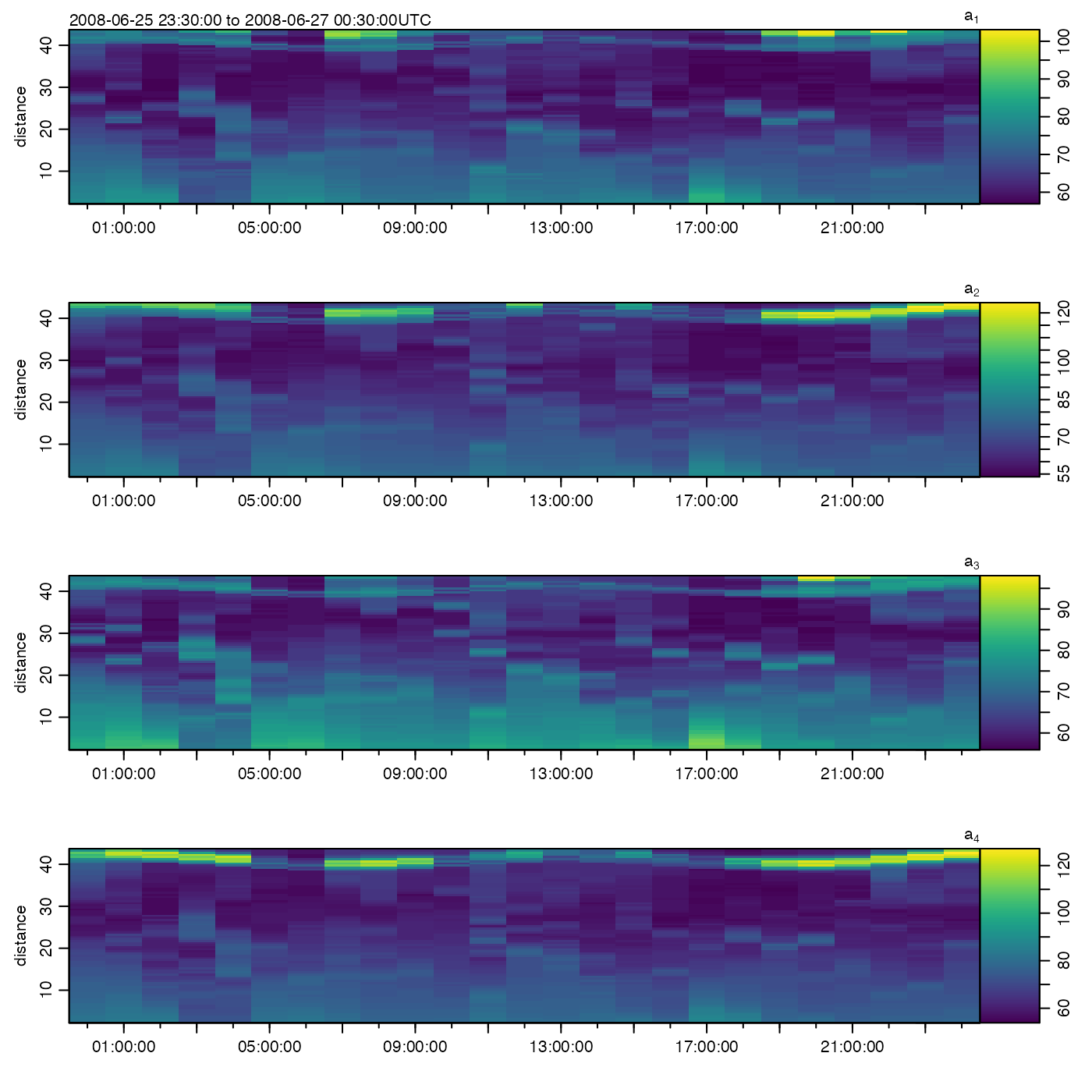beamUnspreadAdp(
x,
count2db = c(0.45, 0.45, 0.45, 0.45),
asMatrix = FALSE,
debug = getOption("oceDebug")
)

## Arguments

x

count2db

a set of coefficients, one per beam, to convert from beam echo intensity to decibels.

asMatrix

a boolean that indicates whether to return a numeric matrix, as opposed to returning an updated object (in which the matrix is cast to a raw value).

debug

an integer specifying whether debugging information is to be printed during the processing. This is a general parameter that is used by many oce functions. Generally, setting debug=0 turns off the printing, while higher values suggest that more information be printed. If one function calls another, it usually reduces the value of debug first, so that a user can often obtain deeper debugging by specifying higher debug values.

## Details

First, beam echo intensity is converted from counts to decibels, by multiplying by count2db. Then, the signal decrease owing to spherical spreading is compensated for by adding the term $$20\log10(r)$$, where $$r$$ is the distance from the sensor head to the water from which scattering is occurring. $$r$$ is given by x[["distance"]].

Other things related to adp data: [[,adp-method, [[<-,adp-method, ad2cpCodeToName(), ad2cpHeaderValue(), adp-class, adpAd2cpFileTrim(), adpConvertRawToNumeric(), adpEnsembleAverage(), adpFlagPastBoundary(), adpRdiFileTrim(), adp_rdi.000, adp, applyMagneticDeclination,adp-method, as.adp(), beamName(), beamToXyzAdpAD2CP(), beamToXyzAdp(), beamToXyzAdv(), beamToXyz(), binmapAdp(), enuToOtherAdp(), enuToOther(), handleFlags,adp-method, is.ad2cp(), plot,adp-method, read.adp.ad2cp(), read.adp.nortek(), read.adp.rdi(), read.adp.sontek.serial(), read.adp.sontek(), read.adp(), read.aquadoppHR(), read.aquadoppProfiler(), read.aquadopp(), rotateAboutZ(), setFlags,adp-method, subset,adp-method, subtractBottomVelocity(), summary,adp-method, toEnuAdp(), toEnu(), velocityStatistics(), xyzToEnuAdpAD2CP(), xyzToEnuAdp(), xyzToEnu()

Dan Kelley

## Examples

library(oce)
plot(adp, which=5) # beam 1 echo intensityplot(adp.att, which=5) # beam 1 echo intensity# Profiles
par(mar=c(4, 4, 1, 1))
a <- adp[["a", "numeric"]]             # second arg yields matrix return value